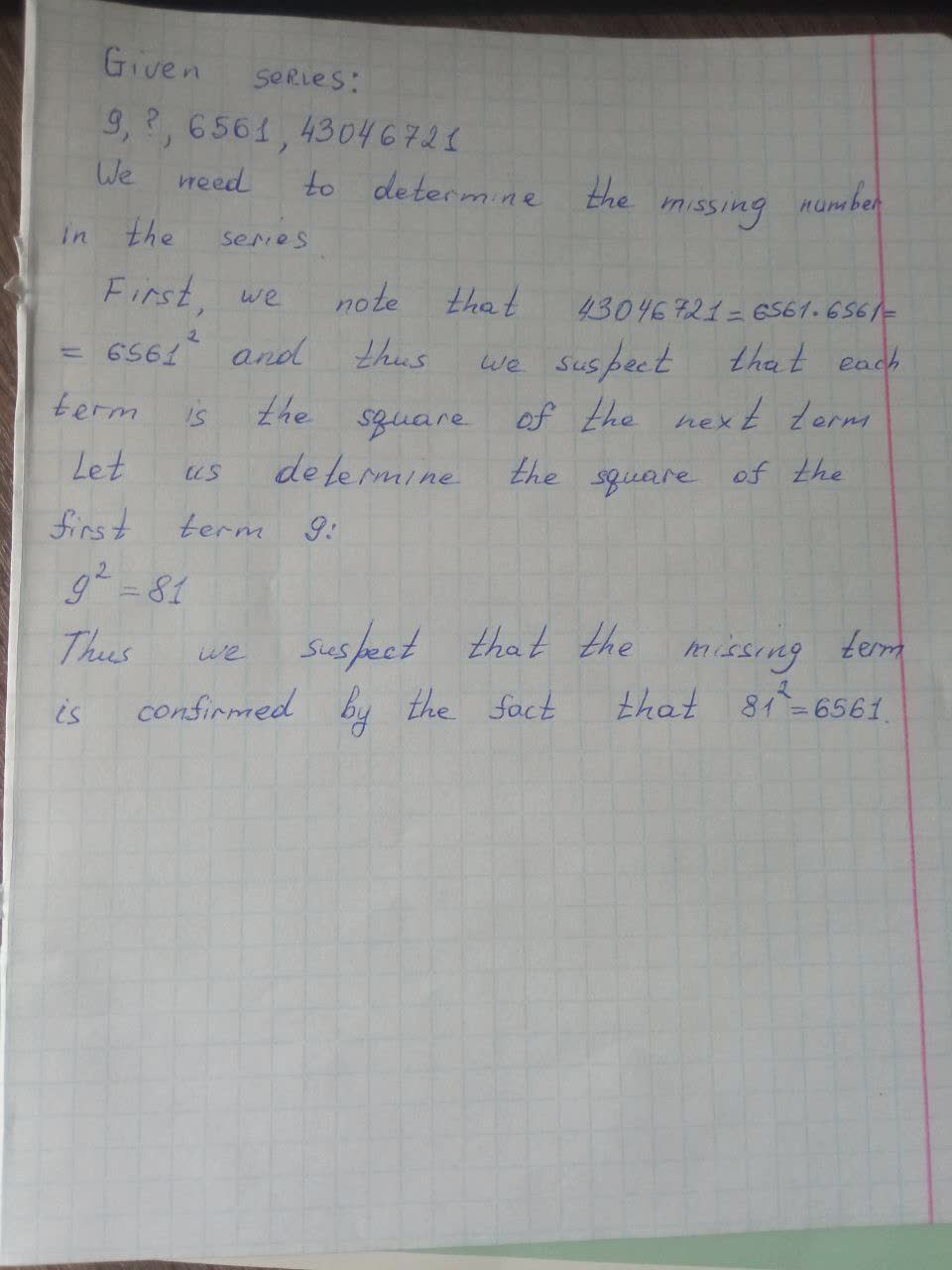# Missing number in the series 9, ____, 6561, 43046721 is: 81 25 62 31 18amanf 2021-06-03 Answered

Missing number in the series is:

You can still ask an expert for help

• Questions are typically answered in as fast as 30 minutes

Solve your problem for the price of one coffee

• Math expert for every subject
• Pay only if we can solve itLaaibah Pitt
Here is a photo, the answer is on it:###### Not exactly what you’re looking for?question2answer

Given series: 9,2, 6561, 43046721
We need to determine the missing number in the series.
First, we note that $43046721=6561\cdot 6561={6561}^{2}$ and thus we suspect that each term is the square of the next term.
Let us determine the square of the first term 9:
${9}^{2}=81$
Thus we suspect that the missing term is 81, which is confirmed by the fact that ${81}^{2}—6561$.